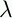September 22, 1999 Perl and the Lambda Calculus Slide #34

# Building an Interpreter

• Why write a parser and an interpreter?

• In Perl:

•x.E => sub { my \$x = shift; E }

• (E F) => \$E->(\$F)

• For example:

```        # TRUE =pq.p
\$TRUE = sub { my \$p = shift;
sub { my \$q = shift;
\$p
};
};
```
• We can use this to try out the abstract stuff from the previous slides

 NextCopyright © 1999 M-J. Dominus